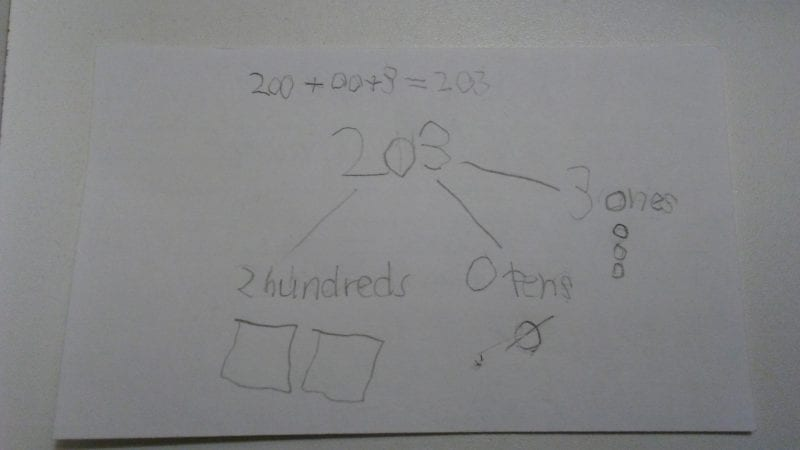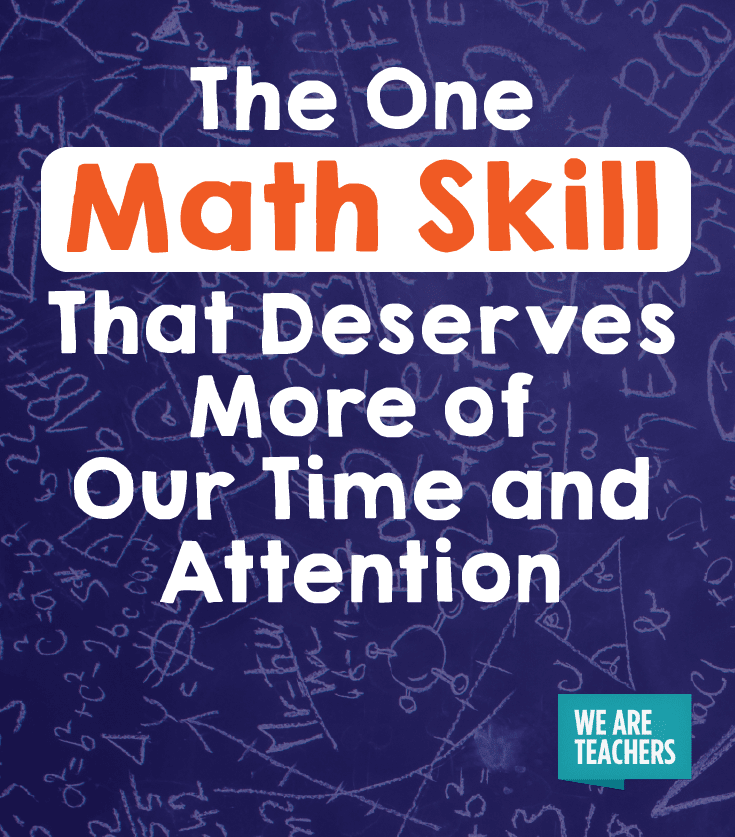# The One Math Skill That Deserves More of Our Time and Attention

We don’t give enough love to expanded form.

Certain math skills receive most of the attention: addition, subtraction, multiplication, division. Fractions are always in the spotlight. There is one skill, though, that deserves more emphasis in our math classrooms: expanded form.

Expanded form is an alternate way for students to write a given number, focusing on the value of each digit. For example, 456 in expanded form is 400 + 50 + 6.

Expanded form is great way to reinforce the concept of place value. Yet it is often taught at the beginning of the year and never returned to again. I believe this is a mistake, and here’s why.

## Those Zeroes Are Worth More Than You Think

Often, young students struggle with the meaning of zero in three- and four-digit numbers. For example, many first graders will write 102 as 1002, combining 100 and 2 into a four-digit number. They know 100 means one hundred, but not that it means 1 hundreds and 0 tens and 0 ones. This is why they don’t understand that they need to replace the 0 in the ones place with 2.

Similarly, middle grade students can struggle to grasp the value of larger numbers, especially as numbers grow in size and abstraction.

Expanded form asks students to split numbers into parts, allowing students to see how digits like 0, 1, 2 and 3 come together to form multi-digit numbers with varying values. 120, 301, and 213 represent just a few of the numbers than can be made with 0, 1, 2 and 3.

One way to make sure students get the most out of expanded form is to also have them label and draw what each of the digits in a number represents.

In the example below, my second grade student split the number 203 into 2 hundreds, 0 tens and 3 ones and had her draw a picture of each. She also wrote out 200 + 00 + 3.

Often students are told they don’t need bother with labelling the 0 tens or writing out 00. I would argue that zeroes are just as important as the non-zero digits. While a zero might signify that you don’t “have” any tens or ones, it definitely has meaning when it comes to place value. Think of the difference between \$102 and \$10,002. Zeroes matter!## Don’t Abandon Expanded Form After the Unit 1 Test

Expanded form is too valuable to stop using after the place value unit ends. Two areas where expanded form supports increased understanding is with addition and decimals.

For addition, ask students to rewrite 34 + 128 in expanded form as 30 + 4  and 100 + 20 + 8 and illustrate the problem by drawing small circles for ones, rods for tens and squares for hundreds. Students benefit from using expanded form here because the emphasis remains on the place value of the numbers they are working with. The pictorial representation provides scaffolding for students who need something to help them count the sum.

You can also return to expanded form when teaching decimals. 4.72 rewritten as 4 + 0.7 + 0.02 highlights the value of each digit as students begin working with tenths and hundredths. Again, using visuals here will only strengthen student conceptualization of what it means to have two hundredths.

Of course, this is only the beginning of how expanded form can be used throughout the year. What are some of the ways you use expanded form in your math classroom?## You Might Also Like